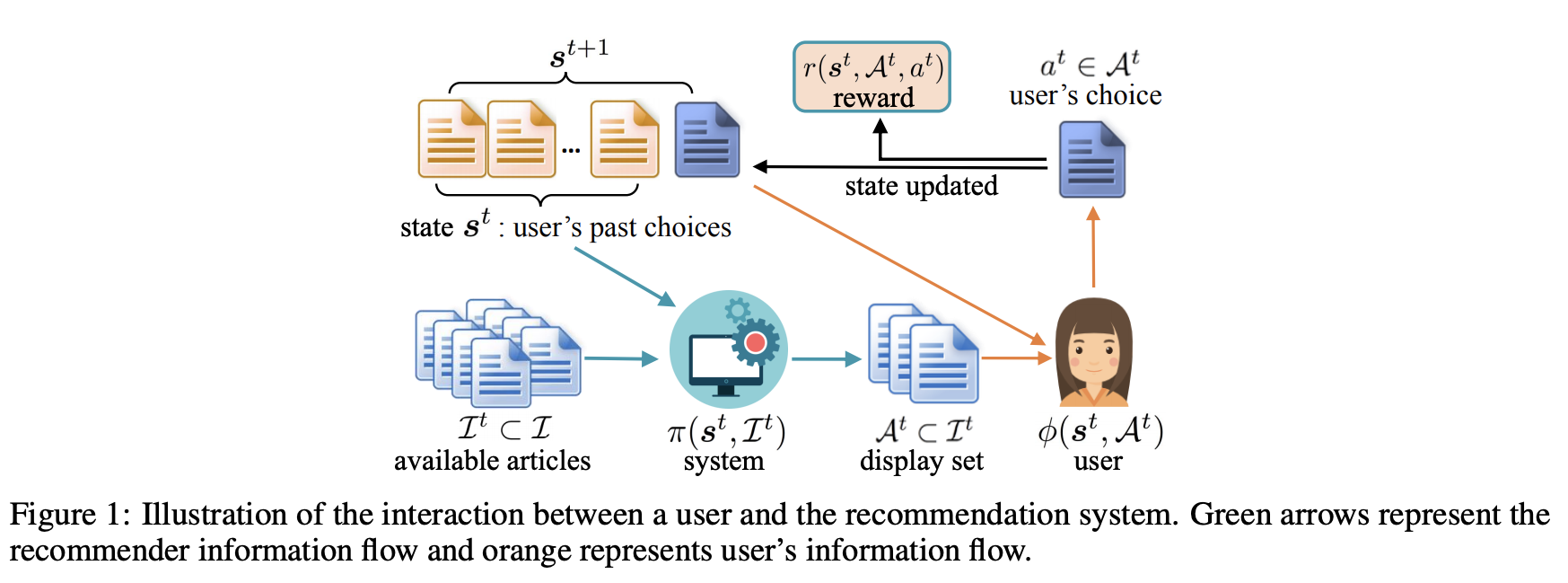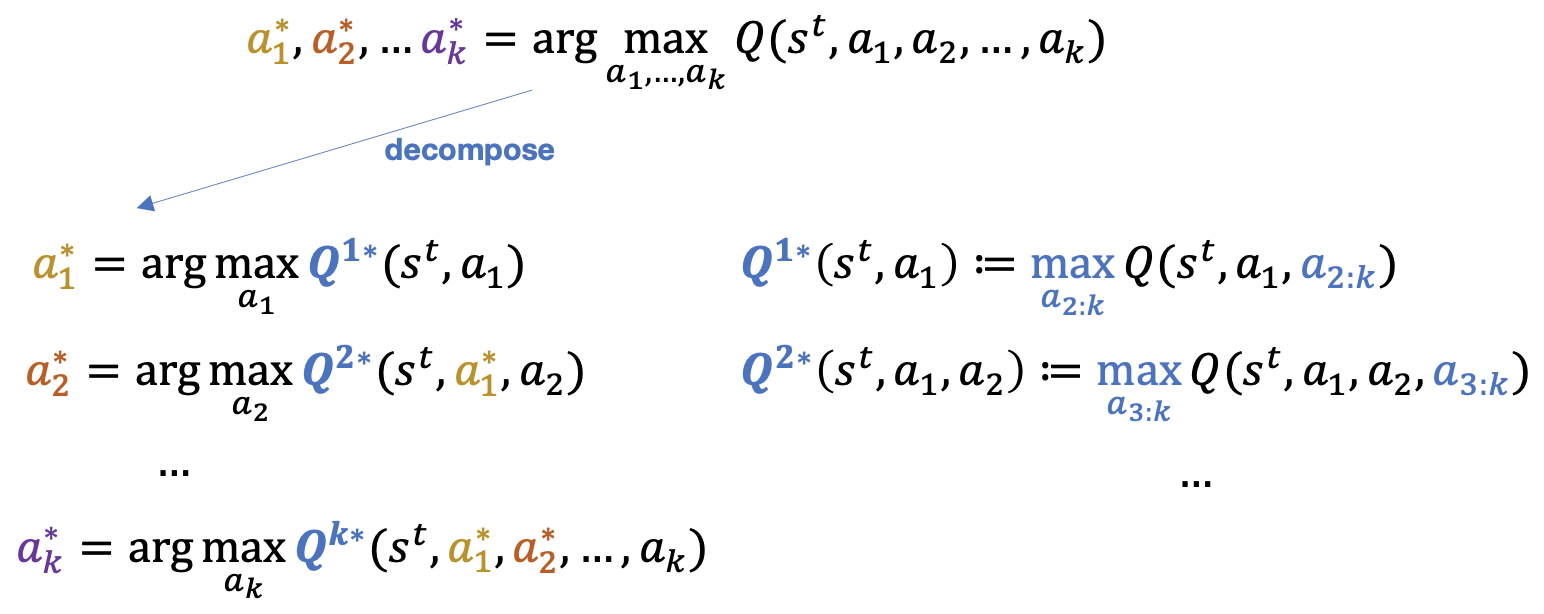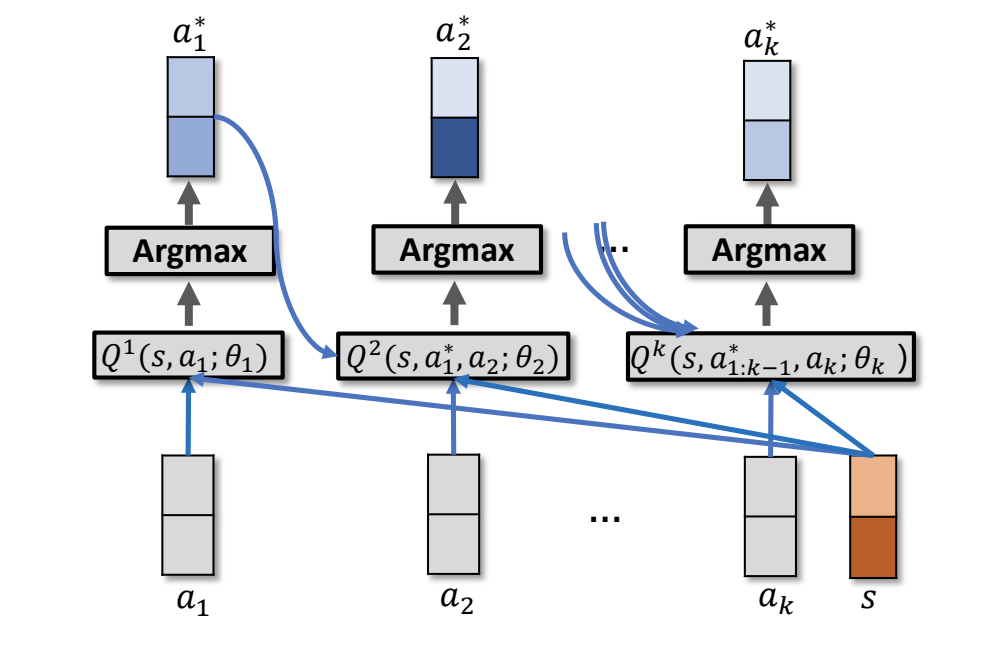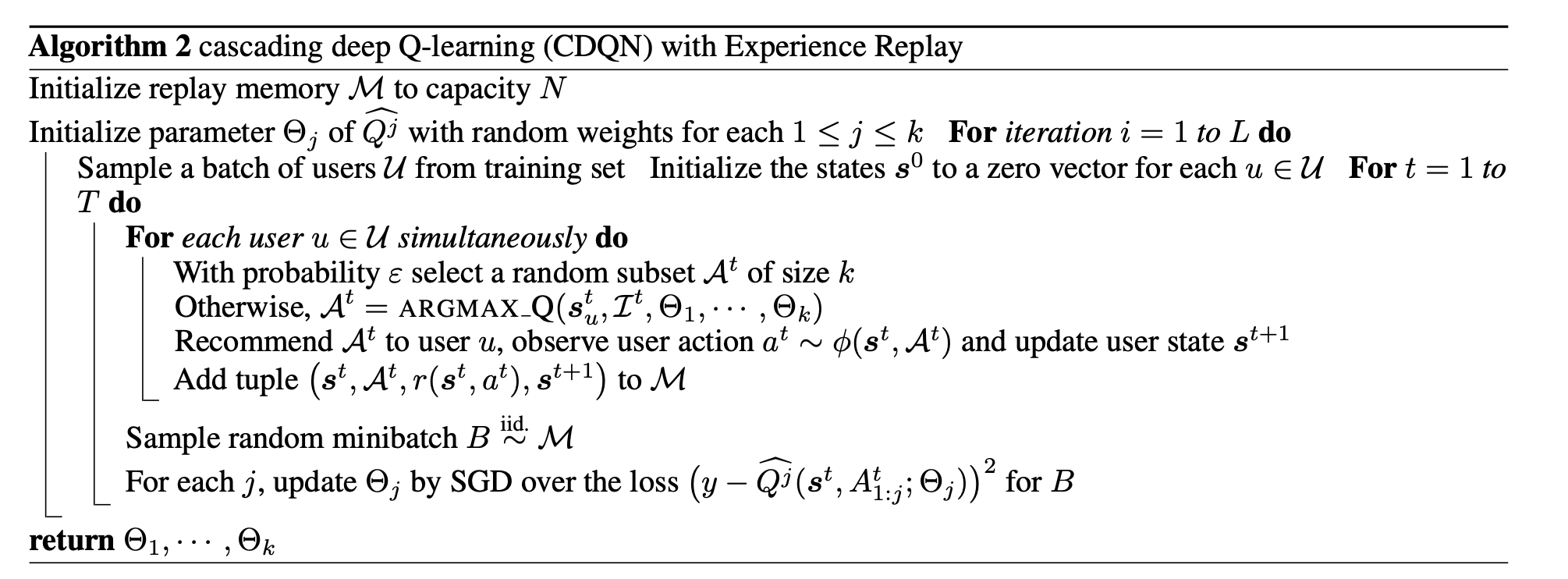To Top

## GAN-CDQN

Generative Adversarial User Model for Reinforcement Learning Based Recommendation System

## 简介

RL在推荐场景中有以下问题：

• 首先，驱动用户行为的兴趣点（奖励函数）一般是未知的，但它对于 RL 算法的使用来说至关重要。在用于推荐系统的现有RL算法中，奖励函数一般是手动设计的（例如用 ±1 表示点击或不点击），这可能无法反映出用户对不同项目的偏好如何(如Deep Reinforcement Learning for Page-wise Recommendations)。
• 其次，无模型RL一般都需要和环境（在线用户）进行大量的交互才能学到良好的策略。但这在推荐系统设置中是不切实际的。如果推荐看起来比较随机或者推荐结果不符合在线用户兴趣，他会很快放弃!!这一服务。

• 开发了生成对抗学习（GAN）方法来对用户行为动态性(dynamics)建模，并recover奖励函数。可以通过联合的minimax优化算法同时评估这两个组件。该方法的优势在于：
• 可以得到更predictive(可预测的？)的用户模型，而且可以用与用户模型一致的方法学习奖励函数
• 相较于手动设计的简单奖励函数，从用户行为中学习到的奖励函数有利于后面的强化学习
• 学习到的用户模型使研究者能够为新用户执行基于模型的RL和在线适应从而实现更好的结果。## Setting和RL Formulation

setting：给用户展示了$$k$$个item，然后他点了1个或者0个，然后展示后$$k$$个item。

$\pi^{*}=\underset{\pi\left(\boldsymbol{s}^{t}, \mathcal{I}^{t}\right)}{\arg \max } \mathbb{E}\left[\sum_{t=0}^{\infty} \gamma^{t} r\left(\boldsymbol{s}^{t}, a^{t}\right)\right]$

• 环境：在推荐的每一页的$$k$$个item中可以点击其中一个的用户
• 状态$$\boldsymbol{s}^{t} \in \mathcal{S}$$：用户历史点击的一个有序序列
• 动作$$\mathcal{A}^{t} \in\left(\begin{array}{c}{\mathcal{I}^{t}} \\ {k}\end{array}\right)$$：推荐系统从$$\mathcal{I}^{t}$$个候选中选择$$k$$个候选的子集。其中，$$\left(\begin{array}{c}{\mathcal{I}^{t}} \\ {k}\end{array}\right)$$表示$$\mathcal{I}^{t}$$中的所有的$$k$$元素子集。而$$\mathcal{I}^{t} \subset \mathcal{I}$$是所有候选$$\mathcal{I}$$在时间步$$t$$的候选子集。
• 状态转移$$P\left(\cdot | s^{t}, \mathcal{A}^{t}\right) : \mathcal{S} \times\left(\begin{array}{l}{\mathcal{I}} \\ {k}\end{array}\right) \mapsto \mathcal{P}(\mathcal{S})$$：给定状态$$\mathcal{s}^{t}$$，以及展示的集合$$\mathcal{A}^{t}$$的情况下，转移到状态$$\boldsymbol{s}^{t+1}$$的转移概率。等价于下文提到的在用户行为上的分布$$\phi\left(s^{t}, \mathcal{A}^{t}\right)$$
• 奖励函数$$r\left(\boldsymbol{s}^{t}, \mathcal{A}^{t}, a^{t}\right) : \mathcal{S} \times\left(\begin{array}{l}{\mathcal{I}} \\ {k}\end{array}\right) \times \mathcal{I} \mapsto \mathbb{R}$$：用户在状态$$\boldsymbol{s}^{t}$$下，采用动作$$a^{t} \in \mathcal{A}^{t}$$得到的回报。在这里假设推荐系统得到的回报和用户得到的回报一样，所以长期回报也是一样。
• 策略$$\mathcal{A}^{t} \sim \pi\left(s^{t}, \mathcal{I}^{t}\right) : \mathcal{S} \times 2^{\mathcal{I}} \mapsto \mathcal{P}\left(\left(\begin{array}{c}{\mathcal{I}_{k}} \\ {k}\end{array}\right)\right)$$：在用户状态$$\mathcal{s}^{t}$$下，从集合$$\mathcal{I}^{t}$$中选择子集$$\mathcal{A}^{t}$$进行展示的概率。

• 环境状态状态转移用户有关，
• 行为策略推荐系统有关。
• 回报推荐系统用户均有关。

reward函数和transition都是未知的，可以从数据中学习。只要这两个学好了，那么就可以通过使用例如Q-learrning等算法，不断地对模型进行query，来估计上文提到的最优的策略$$\pi^{*}=\underset{\pi\left(\boldsymbol{s}^{t}, \mathcal{I}^{t}\right)}{\arg \max } \mathbb{E}\left[\sum_{t=0}^{\infty} \gamma^{t} r\left(\boldsymbol{s}^{t}, a^{t}\right)\right]$$

## Generative Adversarial User Model

### User Behavior As Reward Maximization

• 用户不是消极的(passive)。当用户看到展现的$$k$$个item时，会做出令他自己的回报最大的决定。回报$$r$$意味着这个用户对这个item有多感兴趣或者多满意。而如果他都不感兴趣，可以选择都不点。
• reward不仅和当前这个被选择的item有关，也和用户的历史有关。例如，一个用户听了a的某一首歌，可能他会对a的其他歌也感兴趣；而如果他听了很多a的歌，可能他也会感到厌倦了。这些都是和personal experience有关的。

$\phi^{*}\left(\boldsymbol{s}^{t}, \mathcal{A}^{t}\right)=\arg \max _{\phi \in \Delta^{k-1}} \mathbb{E}_{\phi}\left[r\left(\boldsymbol{s}^{t}, a^{t}\right)\right]-R(\phi) / \eta$

• $$\Delta^{k-1}$$是probability simplex，也就是概率单纯形，参考https://juejin.im/entry/58e09c2cda2f60005fcd5573，简单理解好像。。就是$$k$$个元素，和为1，所以可以看成是一个概率分布。
• $$R(\phi)$$是一个凸的正则函数。
• $$\eta$$能控制正则化的强度

$\phi^{*}\left(\boldsymbol{s}^{t}, \mathcal{A}^{t}\right)_{i}=\exp \left(\eta r\left(\boldsymbol{s}^{t}, a_{i}\right)\right) / \sum_{a_{j} \in \mathcal{A}^{t}} \exp \left(\eta r\left(\boldsymbol{s}^{t}, a_{j}\right)\right)$

$a^{t}=\arg \max _{a \in \mathcal{A}^{t}} \eta r\left(\boldsymbol{s}^{t}, a\right)+\varepsilon^{t}$

• 其他的正则$$R(\phi)$$也可以用，这样$$\phi^{*}$$$$r$$的关系也会变，也就不一定会有那个逼近的形式了
• 对于用户没有点击任意一个item这种情况，可以看成一直在展现集合$$\mathcal{A}^{t}$$中的一个特殊的item。这个item的feature vector可以都搞成0，或者可以把reward定义成一个常量。

### Model Parameterization

$\boldsymbol{s}^{t}=h\left(\boldsymbol{F}_{*}^{t-m : t-1}\right) :=\operatorname{vec}\left[\sigma\left(\boldsymbol{F}_{*}^{t-m : t-1} \boldsymbol{W}+\boldsymbol{B}\right)\right]$$r\left(\boldsymbol{s}^{t}, a^{t}\right) :=\boldsymbol{v}^{\top} \sigma\left(\boldsymbol{V}\left[\begin{array}{c}{\boldsymbol{s}^{t}} \\ {\boldsymbol{f}_{a^{t}}^{t}}\end{array}\right]+\boldsymbol{b}\right)$

$\phi\left(s, \mathcal{A}^{t}\right) \propto \exp \left(\boldsymbol{v}^{\prime \top} \sigma\left(\boldsymbol{V}^{\prime}\left[\begin{array}{c}{\boldsymbol{s}^{t}} \\ {\boldsymbol{f}_{a^{t}}^{t}}\end{array}\right]+\boldsymbol{b}^{\prime}\right)\right)$

### Generative Adversarial Training

• $$\phi$$可以看做是一个generator。基于用户的历史，产生用户的下一个行为。参数是$$\alpha$$，要让把假的当成真实最大，所以在下面的式子里，需要$$\alpha$$最大！
• $$r$$可以看做是一个discriminator，试图分辨出用户的真实行为与generator产生的用户行为。参数是$$\theta$$，要让把真实的当成真实最大，而下面的式子第二项前有个负号，所以要$$\theta$$最小。。

\begin{aligned} \min _{\theta} \max _{\alpha}\left(\mathbb{E}_{\phi_{\alpha}}\right.&\left[\sum_{t=1}^{T} r_{\theta}\left(\boldsymbol{s}_{\text {true}}^{t}, a^{t}\right)\right]-R\left(\phi_{\alpha}\right) / \eta ) -\sum_{t=1}^{T} r_{\theta}\left(\boldsymbol{s}_{\text {true}}^{t}, a_{\text {true}}^{t}\right) \end{aligned}

$\left\{\begin{array}{l}{\alpha \leftarrow \alpha+\gamma_{1} \nabla_{\alpha} \mathbb{E}_{\phi_{\alpha}}\left[\sum_{t=1}^{T} r_{\theta}\right]-\gamma_{1} \nabla_{\alpha} R\left(\phi_{\alpha}\right) / \eta} \\ {\theta \leftarrow \theta-\gamma_{2} \mathbb{E}_{\phi_{\alpha}}\left[\sum_{t=1}^{T} \nabla_{\theta} r_{\theta}\right]+\gamma_{2} \sum_{t=1}^{T} \nabla_{\theta} r_{\theta}}\end{array}\right.$

$\max _{\theta \in \Theta} \prod_{t=1}^{T} \frac{\exp \left(\eta r_{\theta}\left(s_{t r u e}^{t}, a_{t r u e}^{t}\right)\right)}{\sum_{a^{t} \in \mathcal{A}^{t}} \exp \left(\eta r_{\theta}\left(s_{t r u e}^{t}, a^{t}\right)\right)}$

## Cascading RL Policy for Recommendation

• 在combinatorial action space这个空间上的可能的很高的计算复杂度
• 对某种item组合的长期reward(Q)的预估也需要一个复杂度较高的框架

$Q^{*}\left(s^{t}, \mathcal{A}^{t}\right)=\mathbb{E}\left[r\left(s^{t}, \mathcal{A}^{t}, a^{t}\right)+\gamma \max _{\mathcal{A}^{\prime} \subset \mathcal{I}} Q^{*}\left(s^{t+1}, \mathcal{A}^{\prime}\right)\right], a^{t} \in \mathcal{A}^{t}$

$\pi^{*}\left(\boldsymbol{s}^{t}, \mathcal{I}^{t}\right)=\arg \max _{\mathcal{A}^{t} \subset \mathcal{I}^{t}} Q^{*}\left(\boldsymbol{s}^{t}, \mathcal{A}^{t}\right)$

• 输入$$s$$$$a_1$$，找到使得$$Q^{1*}(s,a_1)$$最大的动作$$a_1^*$$
• 输入$$s$$$$a_1^*$$还有$$a_2$$，找到使得$$Q^{2*}(s,a_1^*,a_2)$$最大的动作$$a_2^*$$
• 输入$$s$$$$a_{1:k-1}^*$$还有$$a_k$$，找到使得$$Q^{k*}(s,a_{1:k-1}^*,a_k)$$最大的动作$$a_k^*$$

• 第一步的$$Q^{1*}(s,a_1)$$可以看成是$$Q^*(s,a_{1:k})$$当动作取$$a_{2:k}$$的时候取得最大值
• 第二步的$$Q^{2*}(s,a_1^*,a_2)$$可以看成是$$Q^*(s,a_{1:k})$$当动作取$$a_{3:k}$$的时候取得最大值
• 第k步的$$Q^{k*}(s,a_{1:k-1}^*,a_1)$$可以看成是$$Q^*(s,a_{1:k})$$

\begin{array}{l}{\text { Cascading Q-Networks: }} \\ {\qquad \begin{aligned} a_{1}^{*} &=\arg \max _{a_{1}}\left\{Q^{1 *}\left(s, a_{1}\right):=\max _{a_{2: k}} Q^{*}\left(s, a_{1: k}\right)\right\} \\ a_{2}^{*} &=\arg \max _{a_{2}}\left\{Q^{2 *}\left(s, a_{1}^{*}, a_{2}\right):=\max _{a_{3: k}} Q^{*}\left(s, a_{1: k}\right)\right\} \\ \cdots & \\ a_{k}^{*} &=\arg \max _{a_{k}}\left\{Q^{k *}\left(s, a_{1: k-1}^{*}, a_{k}\right):=Q^{*}\left(s, a_{1: k}\right)\right\} \end{aligned}}\end{array}### Parameterization and Estimation

$\boldsymbol{q}_{j}^{\top} \sigma\left(\boldsymbol{L}_{j}\left[\boldsymbol{s}^{\top}, \boldsymbol{f}_{a_{i}}^{\top}, \ldots, \boldsymbol{f}_{a_{j-1}^{\prime}}^{\top}, \boldsymbol{f}_{a_{j}}^{\top}\right]^{\top}+\boldsymbol{c}_{j}\right), \forall j$

$\boldsymbol{s}^{t}:=h\left(\boldsymbol{F}_{*}^{1: t-1}:=\left[\boldsymbol{f}_{*}^{1}, \cdots, \boldsymbol{f}_{*}^{t-1}\right]\right)$

$\boldsymbol{s}^{t}=h\left(\boldsymbol{F}_{*}^{t-m: t-1}\right):=\operatorname{vec}\left[\sigma\left(\boldsymbol{F}_{*}^{t-m: t-1} \boldsymbol{W}+\boldsymbol{B}\right)\right]$

$Q^{j *}\left(s, a_{1}^{*}, \cdots, a_{j}^{*}\right)=Q^{*}\left(s, a_{1}^{*}, \cdots, a_{k}^{*}\right), \quad \forall j$

$\begin{array}{l}{\left(y-Q^{j}\right)^{2}, \text { where }} \\ {y=r\left(s^{t}, \mathcal{A}^{t}, a^{t}\right)+\gamma Q^{k}\left(s^{t+1}, a_{1: k}^{*} ; \Theta_{k}\right), \forall j}\end{array}$comment here..